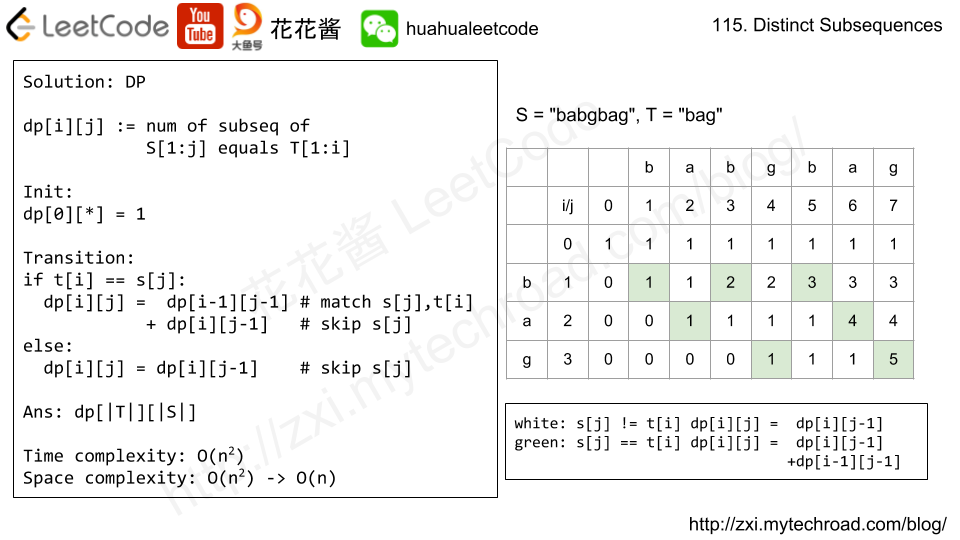# Problem

Given an array of integers A, consider all non-empty subsequences of A.

For any sequence S, let the width of S be the difference between the maximum and minimum element of S.

Return the sum of the widths of all subsequences of A.

As the answer may be very large, return the answer modulo 10^9 + 7.

Example 1:

Input: [2,1,3]
Output: 6
Explanation:
Subsequences are , , , [2,1], [2,3], [1,3], [2,1,3].
The corresponding widths are 0, 0, 0, 1, 1, 2, 2.
The sum of these widths is 6.


Note:

• 1 <= A.length <= 20000
• 1 <= A[i] <= 20000# Solution: Math

Sort the array, for A[i]:

• i numbers <= A[i]. A[i] is the upper bound of 2^i subsequences.
• n – i – 1 numbers >= A[i]. A[i] is the lower bound of 2^(n – i – 1) subsequences.
• A[i] contributes A[i] * 2^i – A[i] * 2^(n – i – 1) to the ans.
$$ans = \sum\limits_{i=0}^{n-1}A_{i}2^{i} – A_{i}2^{n – i – 1} =\sum\limits_{i=0}^{n-1}(A_i – A_{n-i-1})2^{i}$$

Time complexity: O(nlogn)

Space complexity: O(1)

Time complexity: O(n)

Space complexity: O(n)

Counting sort

# Problem

Given a string S and a string T, count the number of distinct subsequences of S which equals T.

A subsequence of a string is a new string which is formed from the original string by deleting some (can be none) of the characters without disturbing the relative positions of the remaining characters. (ie, "ACE" is a subsequence of "ABCDE" while "AEC" is not).

Example 1:

Input: S = "rabbbit", T = "rabbit"
Output: 3
Explanation:  As shown below, there are 3 ways you can generate "rabbit" from S. (The caret symbol ^ means the chosen letters)
rabbbit
^^^^ ^^
rabbbit
^^ ^^^^
rabbbit
^^^ ^^^

Example 2:

Input: S = "babgbag", T = "bag"
Output: 5
Explanation:  As shown below, there are 5 ways you can generate "bag" from S. (The caret symbol ^ means the chosen letters)
babgbag
^^ ^
babgbag
^^ ^
babgbag
^ ^^
babgbag
^ ^^
babgbag
^^^

# Solution: DPTime complexity: O(|s| * |t|)

Space complexity: O(|s| * |t|)

C++

# Related Problems:

## Problem

Given an integer array, your task is to find all the different possible increasing subsequences of the given array, and the length of an increasing subsequence should be at least 2 .

Example:

Input: [4, 6, 7, 7]
Output: [[4, 6], [4, 7], [4, 6, 7], [4, 6, 7, 7], [6, 7], [6, 7, 7], [7,7], [4,7,7]]


Note:

1. The length of the given array will not exceed 15.
2. The range of integer in the given array is [-100,100].
3. The given array may contain duplicates, and two equal integers should also be considered as a special case of increasing sequence.

# Solution: DFS

Time complexity: O(2^n)

Space complexity: O(n)

C++

# Problem

Formally the function should:

Return true if there exists i, j, k
such that arr[i] < arr[j] < arr[k] given 0 ≤ i < j < k ≤ n-1 else return false.

Your algorithm should run in O(n) time complexity and O(1) space complexity.

Examples:
Given [1, 2, 3, 4, 5],
return true.

Given [5, 4, 3, 2, 1],
return false.

Credits:
Special thanks to @DjangoUnchained for adding this problem and creating all test cases.

# Solution: Greedy

Time complexity: O(n)

Space complexity: O(1)

C++

# Problem

https://leetcode.com/problems/longest-palindromic-subsequence/description/

Given a string s, find the longest palindromic subsequence’s length in s. You may assume that the maximum length of s is 1000.

Example 1:
Input:

"bbbab"


Output:

4


One possible longest palindromic subsequence is “bbbb”.

Example 2:
Input:

"cbbd"


Output:

2


One possible longest palindromic subsequence is “bb”.

# Solution: DP

Time complexity: O(n^2)

Space complexity: O(n^2)

C++

Time complexity: O(n^2)

Space complexity: O(n)

C++

Python3

C#

Mission News Theme by Compete Themes.